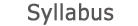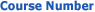0321-1838 Introductory Mathematics for Physicists12019 - 2020View groups
Course description

.Introductory Mathematics for Physicists 1 Syllabus

Instructor

Rennan Barkana

Email :barkana@tau.ac.il

Fall semester

Number of Hours/ Credits

4 lecture + 2 rec.

Mandatory/Elective

Mandatory

Year in program & how often given, if relevant

Year 1, given every year

Course overview – short abstract

This course gives beginning physics students a broad range of mathematical technical skills that are necessary for the physics courses being taken during their first and second years. Emphasis is on problem solving and techniques. Material includes differential and integral calculus of single-variable and multi-variable functions; complex numbers; and ordinary differential equations.

Assignments – 10%

Final exam – 90%

Week-by-week content (approximate)

Week 1: Derivatives: 1-D

Week 2: Taylor series

Week 3: Integrals: 1-D

Week 4: Applications of integrals

Week 5: Multi-dimensional derivatives

Week 6: Linear algebra, Jacobians

Week 7: First-order ordinary differential equations: Intro

Week 8: Techniques for solving 1'st order ODE's

Week 9: Multi-dimensional integrals

Week 10: Applications of 2-D and 3-D integrals

Week 11: Complex numbers: Intro

Week 12: Euler's formula, polynomials

Week 13: Second-order ordinary differential equations

Required text: None

accessibility declaration